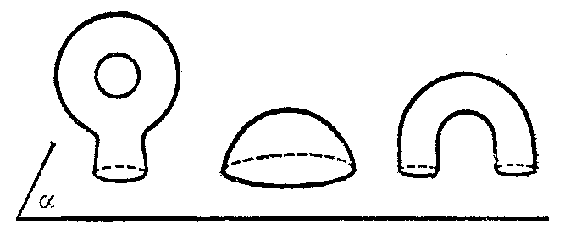##### Actions

A generalization of a surface of negative curvature (cf. Negative curvature, surface of). Let $M$ be a surface in the $3$- dimensional Euclidean space $E ^ {3}$ defined by an immersion $f: W \rightarrow E ^ {3}$ of a two-dimensional manifold $W$ in $E ^ {3}$. A plane $\alpha$ cuts off a crust from $M$ if among the components of the inverse image of the set $M \setminus \alpha$ in $W$ there is one with a compact closure. The part of $M$ that corresponds to this component is called a crust (see Fig.).Figure: s083080a

The surface $M$ is called a saddle surface if it is impossible to cut off a crust by any plane. Examples of saddle surfaces are a one-sheet hyperboloid, a hyperbolic paraboloid and a ruled surface. For a twice continuously-differentiable surface to be a saddle surface it is necessary and sufficient that at each point of the surface its Gaussian curvature is non-positive. A surface for which all its points are saddle points (cf. Saddle point) is a saddle surface.

A saddle surface that is bounded by a rectifiable contour is, with respect to its intrinsic metric induced by the metric of the space, a two-dimensional manifold of non-positive curvature. A number of properties of surfaces of negative curvature can be generalized to the class of saddle surfaces, but it seems that these surfaces do not form such a natural class of surfaces as do convex surfaces.

How to Cite This Entry: